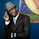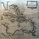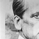656 views
656
Indicator gives the High-Low range of 18 currency pairs. There is a High Ave which is the average of the top four (you may need to adjust which ones are in the average) as well as the bottom four Low Ave. The picture is of a weekly chart with the indicator set at 12 week average of the High-Low range with a .7 (70%) multiplier. When I am looking for week long trades with lots of movement I pick my pairs based on the most actives with a 70% capture of the range as a guide line of when to exit. Sometime when I am not in the mood for volatility I go with the ones in the Low Ave area. Further, when I day trade I set the indicator to a 1Day chart and move the percent to 20% or 30% as a guideline to tell when to get out of a trade.
```//@version=2
study(title="Pairs Range", shorttitle="Pairs Range",precision=0)

l = input(title="Length", type=integer, defval=12, minval=1)

p = input(title="Percent Capture", type=float,defval=.7, minval=.1,step=.05)

p0 = input(title="Other data series", type=symbol,defval="FX_IDC:audjpy")
p1 = input(title="Other data series", type=symbol,defval="FX_IDC:audnzd")
p2 = input(title="Other data series", type=symbol,defval="FX_IDC:audusd")
p3 = input(title="Other data series", type=symbol,defval="FX_IDC:cadjpy")
p17 = input(title="Other data series", type=symbol,defval="FX_IDC:euraud")
p4 = input(title="Other data series", type=symbol,defval="FX_IDC:eurcad")
p5 = input(title="Other data series", type=symbol,defval="FX_IDC:eurgbp")
p6 = input(title="Other data series", type=symbol,defval="FX_IDC:eurjpy")
p7 = input(title="Other data series", type=symbol,defval="FX_IDC:eurnzd")
p8 = input(title="Other data series", type=symbol,defval="FX_IDC:eurusd")
p9 = input(title="Other data series", type=symbol,defval="FX_IDC:gbpaud")
p10 = input(title="Other data series", type=symbol,defval="FX_IDC:gbpjpy")
p11 = input(title="Other data series", type=symbol,defval="FX_IDC:gbpnzd")
p12 = input(title="Other data series", type=symbol,defval="FX_IDC:gbpusd")
p13 = input(title="Other data series", type=symbol,defval="FX_IDC:nzdjpy")
p14 = input(title="Other data series", type=symbol,defval="FX_IDC:nzdusd")
p15 = input(title="Other data series", type=symbol,defval="FX_IDC:usdcad")
p16 = input(title="Other data series", type=symbol,defval="FX_IDC:usdjpy")
// Get the additional data series
s0h= security(p0, period, high)
s1h= security(p1, period, high)
s2h= security(p2, period, high)
s3h= security(p3, period, high)
s4h= security(p4, period, high)
s5h= security(p5, period, high)
s6h= security(p6, period, high)
s7h= security(p7, period, high)
s8h= security(p8, period, high)
s9h= security(p9, period, high)
s10h= security(p10, period, high)
s11h= security(p11, period, high)
s12h= security(p12, period, high)
s13h= security(p13, period, high)
s14h= security(p14, period, high)
s15h= security(p15, period, high)
s16h= security(p16, period, high)
s17h= security(p17, period, high)
s0l= security(p0, period, low)
s1l= security(p1, period, low)
s2l= security(p2, period, low)
s3l= security(p3, period, low)
s4l= security(p4, period, low)
s5l= security(p5, period, low)
s6l= security(p6, period, low)
s7l= security(p7, period, low)
s8l= security(p8, period, low)
s9l= security(p9, period, low)
s10l= security(p10, period, low)
s11l= security(p11, period, low)
s12l= security(p12, period, low)
s13l= security(p13, period, low)
s14l= security(p14, period, low)
s15l= security(p15, period, low)
s16l= security(p16, period, low)
s17l= security(p17, period, low)

r0 = round(sma(s0h-s0l,l)/100*10000*p)
r1 = round(sma(s1h-s1l,l)*10000*p)
r2 = round(sma(s2h-s2l,l)*10000*p)
r3 = round(sma(s3h-s3l,l)/100*10000*p)
r4 = round(sma(s4h-s4l,l)*10000*p)
r5 = round(sma(s5h-s5l,l)*10000*p)
r6 = round(sma(s6h-s6l,l)/100*10000*p)
r7 = round(sma(s7h-s7l,l)*10000*p)
r8 = round(sma(s8h-s8l,l)*10000*p)
r9 = round(sma(s9h-s9l,l)*10000*p)
r10 = round(sma(s10h-s10l,l)/100*10000*p)
r11 = round(sma(s11h-s11l,l)*10000*p)
r12 = round(sma(s12h-s12l,l)*10000*p)
r13 = round(sma(s13h-s13l,l)/100*10000*p)
r14 = round(sma(s14h-s14l,l)*10000*p)
r15 = round(sma(s15h-s15l,l)*10000*p)
r16 = round(sma(s16h-s16l,l)/100*10000*p)
r17 = round(sma(s17h-s17l,l)*10000*p)
plot(r0,color=silver, title="AUDJPY")
plot(r1,color=white,title="AUDNZD")
plot(r2,color=maroon,title="AUDUSD")
plot(r5,color=fuchsia,title="EURGBP")
plot(r6,color=lime,title="EURJPY")
plot(r7,color=olive, title="EURNZD")
plot(r8,color=lime,title="EURUSD")
plot(r9,color=navy, title="GBPAUD")
plot(r10,color=aqua, title="GBPJPY")
plot(r11,color=orange, title="GBPNZD")
plot(r12,color=blue, title="GBPUSD")
plot(r13,color=silver,title="NZDJPY")
plot(r14,color=red,title="NZDUSD")
plot(r16,color=aqua,title="USDJPY")
plot(r17,color=teal, title="EURAUD")
arh = sma((r9+r10+r11+r12)/4,4)
arl = sma((r2+r1+r8+r14)/4,4)
plot(arh,color=yellow,linewidth=5,title="High Ave")
plot(arl,color=yellow,linewidth=5,title="Low Ave")

```I was looking at GBPUSD on the weekly chart. It seems that when GBPUSD was falling in 2008, the indicator shows that GBPUSD is rising to the top of the range. I wonder if something is wrong or that is just how you programmed it. Let me know if you need any modifications. Maybe I can give it a shot.Rocketman
@Rocketman, Good morning Rocketman from EST
GBPUSD does drop hard in 2008. The indicator doesn't show direction of price action. It shows intensity of price action. As GBPUSD is dropping the range of the price action increases. It dropped a great deal as measured by a weekly chart; therefore, the range increases.
Thank you for looking at it.